# Python Tuples Tutorial

## 1- Python Tuples

In Python, Tuples is a sequence of values containing many elements, which is similar to List. Unlike List, Tuples is an immutable data type, all updates on the Tuple create a new entity on the memory.
In order to write a Tuple, you need to put the elements into parentheses ( ) and separated by comma. These elements of the list are indexed with the starting index 0.
tupleExample.py
```
fruitTuple = ("apple", "apricot", "banana","coconut", "lemen")

otherTuple = (100, "one", "two", 3)

print ("Fruit Tuple:")

print (fruitTuple)

print (" --------------------------- ")

print ("Other Tuple:")

print (otherTuple)
```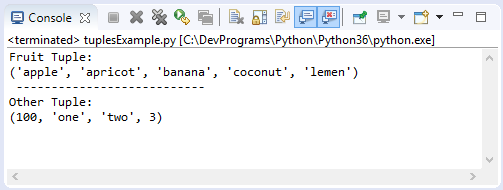## 2- List vs Tuple

See more:
List and Tuple are a sequence of elements. There are some following differences between them:
• Writing a list, you need to use square brackets [] while writing a Tuple, you use parentheses ( ).
```# This is a Tuple.
aTuple = ("apple", "apricot", "banana")

# This is a List.
aList =  ["apple", "apricot", "banana"]
```
• List is a mutable data type, you can use the append() method to add the element to List, or use the remove() method to remove elements from List without creating another "List" entity in memory.
listMemoryTest.py
```list1 =  [1990, 1991, 1992]

print ("list1: ", list1)

# Address of list1 in the memory.
list1Address = hex ( id(list1) )

print ("\n")
print ("Append element 2001 to list1")

# Append a element to list1.
list1.append(2001)

print ("list1 (After append): ", list1)

# Address of list1 in the memory.
list1Address = hex ( id(list1) )

```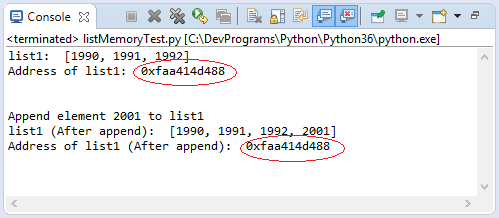• Tuple is an immutable object, it does not have the methods append (), remove (),... like list. You may think that some methods, or operators are used to update Tuple but the fact that it is based on the original Tuple to create a new Tuple.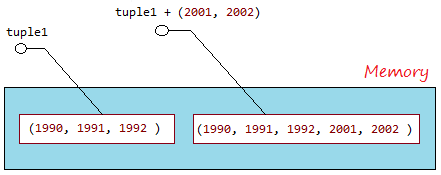tupleMemoryTest.py
```tuple1 =  (1990, 1991, 1992)

# Address of tuple1 in the memory.
tuple1Address = hex ( id(tuple1) )

# Concatenate a tuple to tuple1.
tuple1 = tuple1 + (2001, 2002)

# Address of tuple1 in the memory.
tuple1Address = hex ( id(tuple1) )

```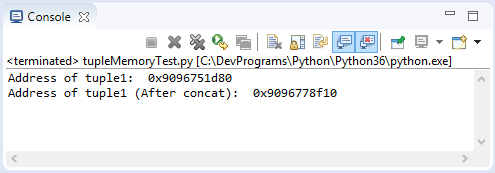## 3- Access the elements of Tuples

### Access the elements

Use the 'for' loop to access the elements of Tuple:
elementAccessExample.py
```
fruits = ("apple", "apricot", "banana", "coconut", "lemen", "plum", "pear")

for fruit in fruits :

print ("Fruit: ", fruit)
```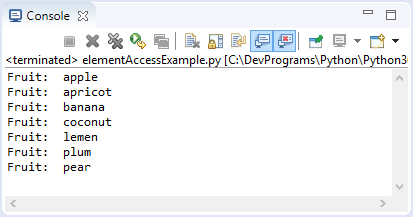### Access via index:

You can also access the elements of Tuple via index. The elements of Tuple are indexed from  left to right, starting at 0.
indexAccessExample.py
```fruits = ("apple", "apricot", "banana", "coconut", "lemen", "plum", "pear")

print ( fruits )

# Element count.
print ("Element count: ", len(fruits) )

for i in range (0, len(fruits) ) :

print ("Element at ", i, "= ", fruits[i] )

# A sub Tuple of elements with index from 1 to 4 (1, 2, 3)
subTuple = fruits[1: 4]

# ('apricot', 'banana', 'coconut')
print ("Sub Tuple [1:4] ", subTuple )
```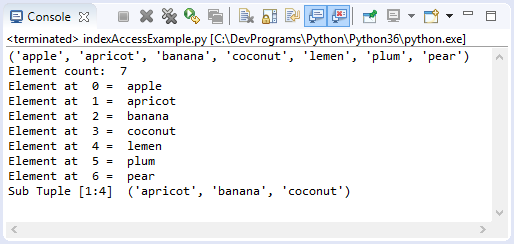You can also access the elements of Tuple via negative index, the elements are indexed from right to left with the values -1, -2,...
indexAccessExample2.py
```fruits = ("apple", "apricot", "banana", "coconut", "lemen", "plum", "pear")

print ( fruits )

print ("Element count: ", len(fruits) )

print ("fruits[-1]: ", fruits[-1])
print ("fruits[-2]: ", fruits[-2])

subTuple1 = fruits[-4: ]

print ("\n")
print ("Sub Tuple fruits[-4: ] ")
print (subTuple1)

subTuple2  = fruits[-4:-2]

print ("\n")
print ("Sub Tuple fruits[-4:-2] ")
print (subTuple2)
```## 4- Update Tuples

Note that Tuple is immutable, so it only has methods or operators to access, or create a new Tuple from the original Tuple.
updateTupleExample.py
```tuple1 = (1990, 1991, 1992)

print ("tuple1: ", tuple1)

print ("Concat (2001, 2002) to tuple1")

tuple2 = tuple1 + (2001, 2002)

print ("tuple2: ", tuple2)

# A sub Tuple, containing elements from index 1 to 4 (1,2,3)
tuple3 = tuple2[1:4]

print ("tuple2[1:4]: ", tuple3)

# A sub Tuple, containing elements from index 1 to the end.
tuple4 = tuple2[1: ]

print ("tuple2[1: ]: ", tuple4)
```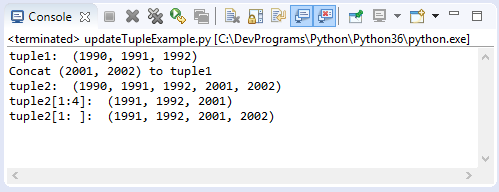## 5- Operators for Tuples

Like String, Tuple has 3 operators including +, * , in.
 Operator Description Example + The operator is used to concatenate 2 Tuples to create a new Tuple (1, 2, 3) + ("One","Two") --> (1, 2, 3, "One", "Two") * The operator is used to concatenate multiple copies of the same Tuple. And create a new Tuple (1, 2) * 3 --> (1, 2, 1, 2, 1, 2) in Check whether an element is in a Tuple, which returns True or False. "Abc" in ("One", "Abc") --> True
tupleOperatorsExample.py
```tuple1 = (1, 2, 3)

tuple2 = ("One", "Two")

print ("tuple1: ", tuple1)
print ("tuple2: ", tuple2)
print ("\n")

tuple12 = tuple1 + tuple2

print ("tuple1 + tuple2: ", tuple12)

tuple2x3 = tuple2 * 3

print ("tuple2 * 3: ", tuple2x3)

hasThree = "Three" in tuple2

print ("'Three' in tuple2? ", hasThree)
```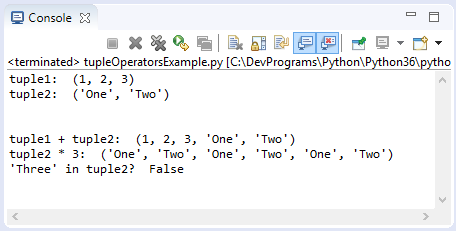## 6- Functions for Tuples

Function Description
cmp(list1, list2) Compares elements of both Tuples. This function was removed from Python3.
len(list) Gives the total length of the Tuple.
max(list) Returns item from the Tuple with max value.
min(list) Returns item from the Tuple with min value.
tuple(seq) Converts a List into Tuple.
tupleFunctionsExample.py
```tuple1 = (1991, 1994, 1992)

tuple2 = (1991, 1994, 2000, 1992)

print ("tuple1: ", tuple1)
print ("tuple2: ", tuple2)

# Return element count.
print ("len(tuple1): ", len(tuple1) )
print ("len(tuple2): ", len(tuple2) )

# Maxinum value in the Tuple.
maxValue = max(tuple1)

print ("Max value of tuple1: ", maxValue)

# Minimum value in the Tuple.
minValue = min(tuple1)

print ("Min value of tuple1: ", minValue)

# (all)
# List
list3 = [2001, 2005, 2012]

print ("list3: ", list3)

# Convert a List to a tuple.
tuple3 = tuple (list3)

print ("tuple3: ", tuple3)
```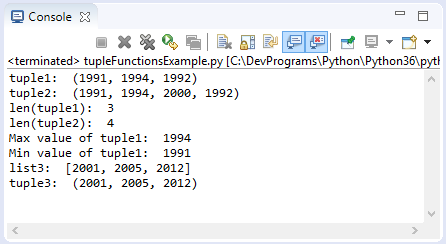See more:

## 7- Methods

Method Description
tuple.count(obj) Returns count of how many times obj occurs in Tuple
tuple.index(obj, [start, [stop]]) Returns the lowest index in Tuple that obj appears. Raises ValueError if the value not found.

If there are parameters start, stop, just search from start to stop index (And not included stop).
Example:
tupleMethodsExample.py
```years =  ( 1990 ,  1991 ,  1993 ,  1991 ,  1993 ,  1993 ,  1993 )

print ("Years: ", years)

print ("\n")

# return number of occurrences of 1993
print ("years.count(1993): ",years.count(1993) )

# Find the index that appears 1993
print ("years.index(1993): ", years.index(1993) )

# Find the index that appears 1993, from index 3
print ("years.index(1993, 3): ", years.index(1993, 3) )

# Find the index that appears 1993, from index 4 to 5 (Not included 6)
print ("years.index(1993, 4, 6): ", years.index(1993, 4, 6) )
```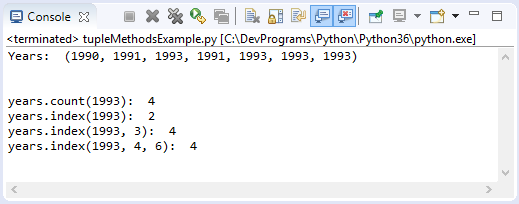See more:

#### View more Tutorials:

Maybe you are interested

These are online courses outside the o7planning website that we introduced, which may include free or discounted courses.

•Learn iPython: The Full Python IDE
•New to Python Automation..?Try Step by Step Python 4 Testers
•Learn Programming in Python With the Power of Animation
•Python Programming Full Course (Basics,OOP,Modules,PyQt)
•Python 3 For Beginner - Object-Oriented Programming
•Complete Python Web Course: Build 8 Python Web Apps
•Web Programming with Python
•Python 2000: Beyond The Basics
•Python GUI : From A-to-Z With 2 Final Projects
•Learning Path: Python Web Development
•Learning iPython Notebook
•Python MySql From Scratch
•Master Computer Vision™ OpenCV4 in Python with Deep Learning
•Learning Path: Python GUI Projects
•Python 3000: Tactical File I/O